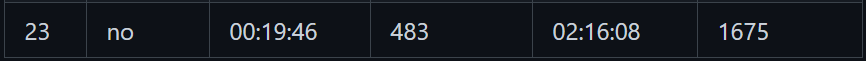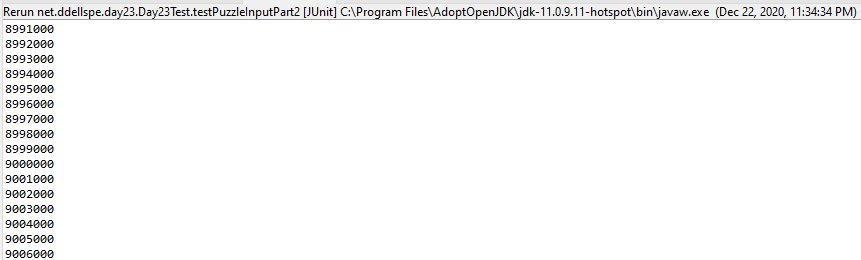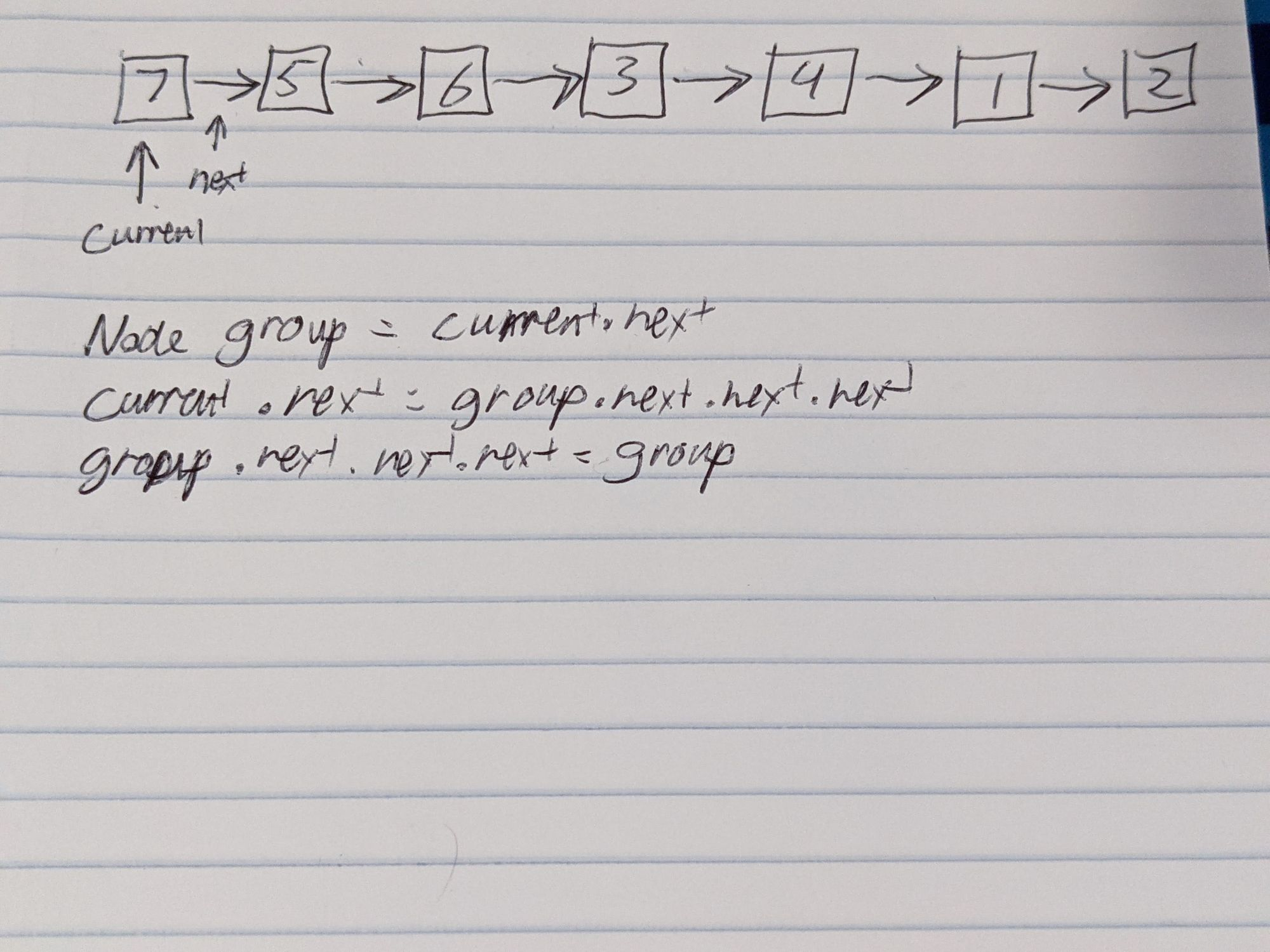# Advent of Code 2020 Day 23

Part two was a different beast, and to be clear, I started it right after part one and the attempt using my part one code to get the part two answer is STILL running (this is after now 10 hours of running) I let it run overnight as I slept, because I was curious if my logic worked to begin with.I learned about linked lists back in 2008 when I took my second programming class in college, Computers in Engineering. We used C structs to implement both a single-linked list and double-linked list (as well as trees and other things). I did find parts of these assignments in my old dropbox folder, I've made a gist of it here (and yes, this is incomplete, but apparently that's all I had stored in this folder). None of this actually matters, but I just thought it was interesting that I had to go ahead and implement something like this yet again with a slightly different situation with respect to how it operates. Linked Lists are fairly straightforward data structures in the programming realm, and kind of match some of the ways that you might consider doing things in a bit more of a relative way. Typically linked lists are used when you're simply shuffling things in a list and today's puzzle was effectively the epitome of simply shuffling items in a list around.

Part one had 100 iterations and really only involved a small set of data to process, so I implemented it with a List and did some manual processing using `subList` to get the next 3 elements and using things like `List.indexOf(Object element)` to figure out the insertion point of data. I ALSO used streams in order to programmatically determine the value to put the 3 obtained values from. This resulted in some very inefficient code, but for 100 iterations and 9 elements in the list, it for sure wasn't really a thought that passed my mind. I ended up in the top 500 submissions (number 483) for part one, which is the best I've ever done for a puzzle submission at this point. I will say, the best part was that I didn't really have to do any debugging to get my answer correct.

Part two was a different beast, and to be clear, I started it right after part one and the attempt using my part one code to get the part two answer is STILL running (this is after now 10 hours of running) I let it run overnight as I slept, because I was curious if my logic worked to begin with. I'm hoping I'll find out soon whether or not that's the case, but I'm honestly not holding my breath at this point. If you're wondering about progress, it's currently 10:15 AM, I started the run at 11:34 PM and I'm nearly to the 9 millionth iteration and we're processing about 1000 values every 4.5 seconds, so with this progress, it SHOULD be done in 75 minutes, but again, I'm not holding my breath with respect to it producing the correct output.Processing as of 10:10 AM of the part 2 solution using my old part one rules. This code no longer exists, but we'll see how it goes.

When I started the processing and it was slow, I knew I needed to look for an alternative. I tried simply changing to the Java LinkedList, but the reality is that while the Java `LinkedList` is implemented AS a "Linked List" as discussed earlier, in order to actually benefit from the actual BENEFITS of Linked Lists, I had to implement my own version. The problem with just the linked list (in this case) though is that by only using the Linked List means for THIS problem, we still need the ability to have random access to the list for insertion. So, in addition to the linked list nodes and their inter-linking, I also created a `HahsMap` to store the reference to the node for each index in order to speed up the processing for these items. In order to develop the data for this, there were 3 steps that I needed to handle.

1. Removing the next 3 values from the list (keeping a reference to them)
2. Finding the next value to move those 3 values to be after
3. Inserting those 3 values after the next value

I used the following data structure for a node in the linked list (btw, this looks eerily familiar to my code from 2008).

``````class Node {
int data;
Node next;
}
``````

So, now I know I can do the following in order to get the group of the next three values out given that `current` is the first node in the list.

``````Node group = current.next;
current.next = group.next.next.next;
group.next.next.next = group;
``````

I drew images to process steps 1 and 3, because I still need to do that some of the time. The images for part 1 are below in the galleryStep 1 operations (in a small example) and the final state

Now the process to determine the next value is somewhat interesting. We know the maximum value in the list (for part two) is 1,000,000 and that if we get to a next value that's in the list of the group that we generated earlier, we have to go to the next lowest value. If we hit that next lowest value it resets to the highest possible value (1,000,000) and so on and so forth. This was done (for me) with a while loop and taking the values of the `group` array into a list for future use.

``````List<Integer> values = Arrays.asList(
group.data,
group.next.data,
group.next.next.data);
int nextValue = current.data - 1;
if(nextValue == 0){
nextValue = 1000000;
}
while(values.contains(nextValue)){
nextValue--;
if(nextValue == 0){
nextValue = 1000000;
}
}
``````

So, now we have `group` which is the 3 data points we need to move, `nextValue` which is the value of the data where we need to insert the `group` and we still have `current` which holds our "head position". The insertion and preparation for the next step is pretty simple, so to continue it on, it's a just an easy procedure. In this case, make note that `nodeMap` contains a key-value entry from key node stored value (`data`) to value Node (the reference to the actual object). So, rather than in our part one solution where we were finding the index to insert at, here, we can actually do some pretty easy operations to insert the list, and then move the current node up a position.

``````group.next.next.next = nodeMap.get(nextValue).next;
nodeMap.get(nextValue).next = group;
current = current.next;
``````

The previous solution required traversal of the list (of 1,000,000 elements) multiple times within the loop.

1. get the `nextValue`
2. get the `nextValue` if you hit 0 as the value
3. find the index of the `nextValue` for insertion

We've now reduced that count to 0, as we're only looking at 3 elements. The real optimization is the fact that we've gone from a triple traversal (`O(n)`) to constant time for this processing. The only difference here is in the map lookup, but since we're leveraging the `HashMap` these lookups are pretty simple as it hashes the key and then it's just a key hash value lookup in the `HashMap` implementation.

In the end, my runtime for this work is now less than 3 seconds for both the sample input as well as the final input (which is good, because they're really doing the same thing here). If I post this before the overnight version completes, I'll update it with how long the run took at the end of the post. If you want to join my Advent of Code leaderboard, feel free to join with the code: `699615-aae0e8af`.

EDIT (2020-12-23 12:07 PM CST):
I just got through the end of the processing, after 43,794.738 seconds (12.165 hours) The result came back, and it was the wrong value.  I think in my work, I remember finding this kind of an issue with the input puzzle and it being caused by accidentally adding a value twice (my loop to extend the range of the values didn't increase the first value by 1 to start from the length + 1.